# Triangles

Here you will learn about triangles, including what a triangle is and how to solve problems involving their sides and their angles.

Students first learn about triangles in kindergarten when they learn how to identify and describe 2D shapes. Students expand their understanding of triangles through elementary and middle school.

## What are triangles?

Triangles are enclosed 2 D shapes with three straight sides, three vertices, and three angles. Triangles are also polygons.

Triangle ABC has 3 sides AB, BC, and AC.

Triangle ABC has 3 vertices, A, B, and C.

Triangle ABC has three angles, Angle A, Angle B, and Angle C.

## Types of triangles

There are different types of triangles that have different properties. Triangles can be grouped by their sides and by their angles.

Classifying triangles by their sidesClassifying triangles by their angles

Scalene Isosceles Equilateral
Triangle Triangle Triangle

Right Acute Obtuse
Triangle Triangle Triangle

Step-by-step guide: Types of triangles

## Equilateral triangles

An equilateral triangle has the following properties,

• The lengths of the sides are all equal.
• The angles are equal measure (60^{\circ}).

The tick marks show that the sides and angles are equal.

An equilateral triangle has 3 lines of symmetry. The lines go from one vertex to the middle of the opposite side.

Step-by-step guide: Equilateral triangles

## Isosceles triangles

An isosceles triangle has the following properties,

• Two side lengths are equal.
• Two equal angle measures are the same.

The two equal angles are called base angles and they are opposite the sides of equal length. The unequal side is known as the base and is opposite the unequal angle.

Isosceles triangles have one line of symmetry.

Step-by-step guide: Isosceles triangles

## Scalene triangles

A scalene triangle has the following properties,

• Side lengths are all different lengths.
• Angles are all different measures.

Scalene triangles have no lines of symmetry.

Step-by-step guide: Scalene triangles

## Right triangles

A right-angled triangle has the following property,

• One right angle

The two sides of the right triangle that meet at the right angle are perpendicular to each other. Line segments that are perpendicular form a right angle.

The longest side of a right-angled triangle is opposite the right-angle. It is called the hypotenuse.

Step-by-step guide: Right triangles

## Acute and obtuse triangles

An acute triangle has three acute angles.

An obtuse triangle has one obtuse angle and the other two angles are acute.

Step-by-step guide: Acute and obtuse triangles

### What are triangles?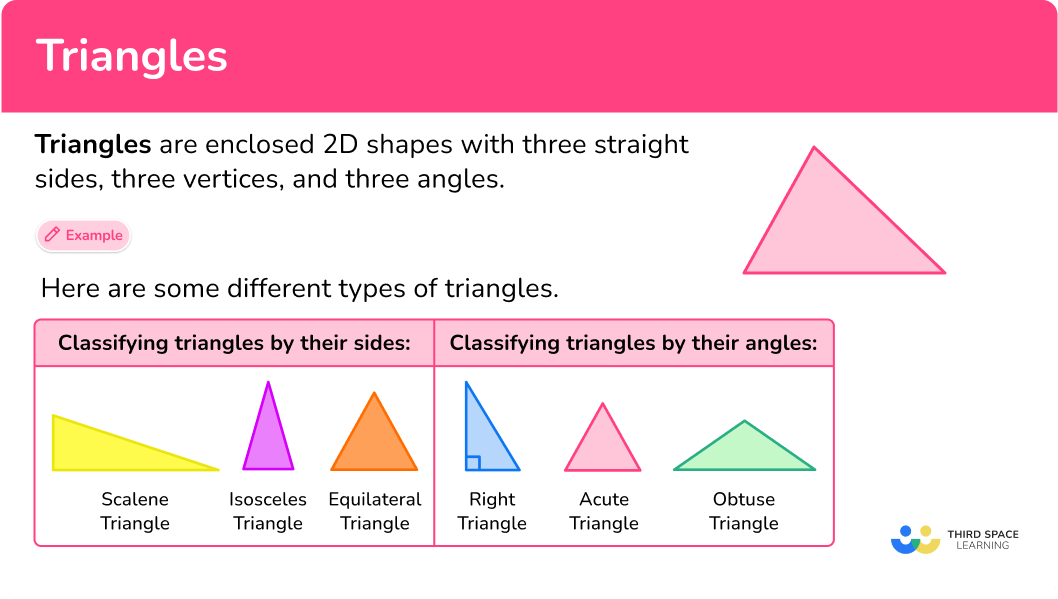## Common Core State Standards

How does this apply to 2nd grade math through 5th grade math?

• Grade 2 – Geometry (2.G.A.1)
Recognize and draw shapes having specified attributes, such as a given number of angles or a given number of equal faces. Identify triangles, quadrilaterals, pentagons, hexagons, and cubes.

• Grade 4 – Geometry (4.G.A.1)
Draw points, lines, line segments, rays, angles (right, acute, obtuse), and perpendicular and parallel lines. Identify these in two-dimensional figures.

• Grade 4 – Geometry (4.G.A.2)
Classify two-dimensional figures based on the presence or absence of parallel or perpendicular lines, or the presence or absence of angles of a specified size. Recognize right triangles as a category, and identify right triangles.

• Grade 4 – Geometry (4.G.A.3)
Recognize a line of symmetry for a two-dimensional figure as a line across the figure such that the figure can be folded along the line into matching parts. Identify line-symmetric figures and draw lines of symmetry.

• Grade 5 – Geometry (5.G.B.3)
Understand that attributes belonging to a category of two-dimensional figures also belong to all subcategories of that category.

## How to answer questions involving triangles

There are a lot of ways to use triangles. For more specific step-by-step guides, check out the individual pages linked in the “What are triangles?” section above or read through the examples below.

In order to solve problems involving triangles:

1. Recall the definition.
2. Explain how the shape fits the definition.

## Triangles examples

### Example 1: identify parts triangles

How many sides, angles, and vertices does the figure below have?

1. Recall the definition.

A triangle is a 2 D shape that has 3 sides, 3 angles, and 3 vertices.

2Explain how the shape fits the definition.

All triangles are polygons that have 3 sides, 3 angles, and 3 vertices.

### Example 2: identify triangles

Identify the triangle as scalene, isosceles, or equilateral.

A scalene triangle has no equal sides.

An isosceles triangle has 2 equal sides.

An equilateral triangle has 3 equal sides.

The triangle has two sides that are 14 \, cm which means they are equal. So the triangle is isosceles.

### Example 3: identify triangles

Identify the triangle as obtuse, acute, or right.

An obtuse triangle has one angle that is greater than 90^{\circ} and less than 180^{\circ}.

An acute triangle has three angles that are greater than 0^{\circ} and less than 90^{\circ}.

A right triangle has one angle that is equal to 90^{\circ}.

The triangle has one right angle because there is the box symbol (which means right angle) at one vertex. So the triangle is a right triangle.

### Example 4: identify triangles

Identify the triangle as scalene, equilateral, or isosceles.

A scalene triangle has no equal sides.

An isosceles triangle has 2 equal sides.

An equilateral triangle has 3 equal sides.

The triangle is marked with three equal sides, so this triangle is an equilateral triangle.

### Example 5: identify triangles

Identify the triangle by its angles and by its sides.

A scalene triangle has no equal sides.

An isosceles triangle has 2 equal sides.

An equilateral triangle has 3 equal sides.

An obtuse triangle has one angle that is greater than 90^{\circ} and less than 180^{\circ}.

An acute triangle has three angles that are greater than 0^{\circ} and less than 90^{\circ}.

A right triangle has one angle that is equal to 90^{\circ}.

The triangle has two sides that equal 11 inches so they are equal.

The triangle has one angle that is greater than 90^{\circ} and less than 180^{\circ}. So the triangle is an isosceles, obtuse triangle.

### Example 6: symmetry in triangles

How many lines of symmetry does the equilateral triangle have?

A line of symmetry is when an object can be divided in half with the two halves being reflections of each other.

An equilateral triangle has 3 lines of symmetry.

### Teaching tips for triangles

• Have students investigate the various types of triangles by measuring sides and angles. This way they can discover the properties for themselves.

• Infuse digital game playing so that students can practice as opposed to giving worksheets.

• Include creative projects such as triangle art projects so that students have opportunity to see the connection between geometry and art.

### Easy mistakes to make

• Mixing up angle facts
For example, confusing acute angles for obtuse angles.

• Mixing up how to group triangles together
For example, there is no such triangle as a scalene, isosceles triangle.

### Practice triangle questions

1. Which of these shapes show an equilateral triangle?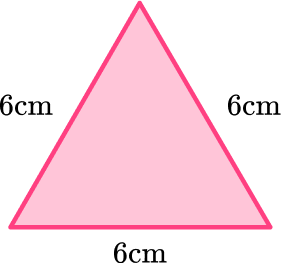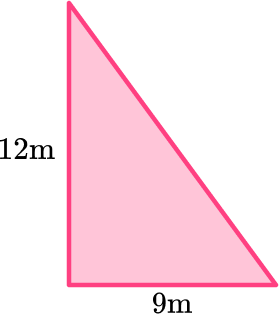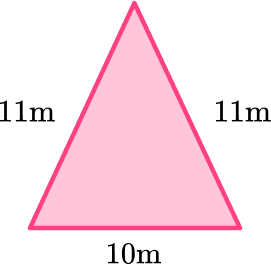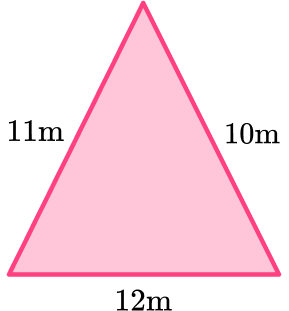An equilateral triangle has three equal sides.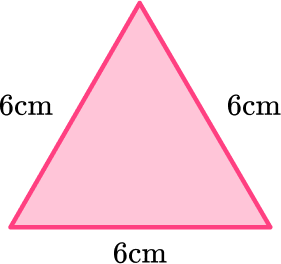This triangle shows all three sides equal to 6 \, cm.

2. The sides of a triangle are 4 \, cm, \, 4 \, cm, and 6 \, cm. What kind of triangle must it be?

Right triangleScalene triangleEquilateral triangleIsosceles triangleA triangle with side measurements of 4 \, cm, \, 4 \, cm, and 6 \, cm has two sides with the same measurement.

So the triangle has two equal sides, which means it is an isosceles triangle.

3. Which of these shapes shows an obtuse triangle?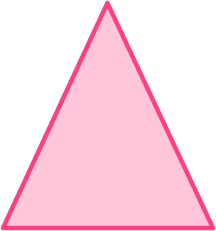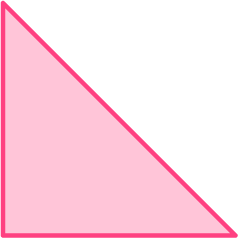An obtuse triangle is a triangle that has one angle that is is greater than 90^{\circ} and less than 180^{\circ}.This is the only triangle that has one angle that is greater than 90^{\circ} and less than 180^{\circ}.

4. Which shape is a right triangle?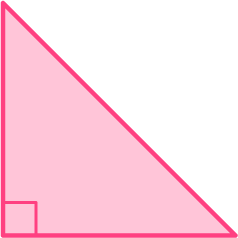A right triangle is a triangle with one right angle. The right angle is symbolized by a square at one of the vertices.This triangle is a right triangle because of the right angle symbol.

5. Which shape is a right triangle and a scalene triangle?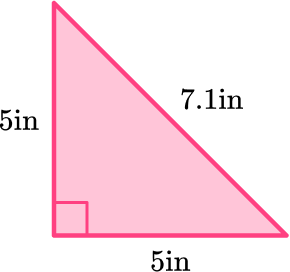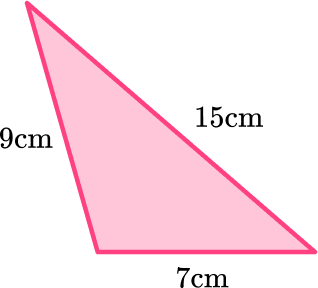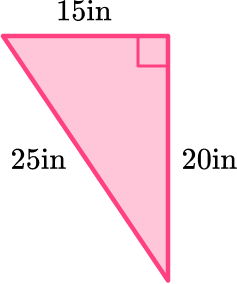A right triangle that is also scalene is a triangle with one right angle and 3 sides that are not equal.This triangle shows the right angle by the square box at one vertex. It also shows the three sides being different lengths.

6. Which triangle has only one line of symmetry?

Scalene triangleEquilateral triangleRight, scalene triangleIsosceles triangleIsosceles triangles have two equal sides. You can draw a line of symmetry down the middle of the triangle, dividing it into two equal halves.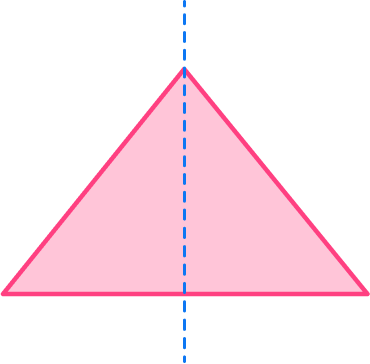## Triangles FAQs

Are there relationships between the angles of the triangle?

Yes, when you get into 8th grade and high school you will learn theorems that explain the relationship of interior angles of triangles. For example, the angle measures of a triangle sum to be 180^{\circ} .

What is the exterior angle of a triangle?

The exterior angle of a triangle is the angle that is supplementary to one of the interior angles.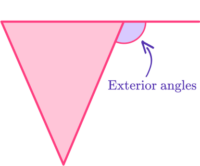Are there relationships between the sides of the triangles?

Yes, when you get into 8th grade math and high school, you will learn theorems that explain relationships between the sides of triangles. For example, you will learn that the sum of the two shorter sides of a triangle must be greater than the third side of the triangle.

What is trigonometry?

Trigonometry is the study of triangles. You will learn trigonometry and right triangle trigonometry in high school, where you will learn the sine, cosine, and tangent ratios.

What does congruent mean?

Congruent means exactly the same shape and size. In geometry, the word “congruent” is often used for “equal” because you are comparing shapes.

What is euclidean geometry?

Euclidean geometry is the study of plane and solid figures and is based on the work of Euclid.

What is Heron’s formula?

It is a formula you will use in high school to find the area of a triangle without knowing the height of the triangle.

## Still stuck?

At Third Space Learning, we specialize in helping teachers and school leaders to provide personalized math support for more of their students through high-quality, online one-on-one math tutoring delivered by subject experts.

Each week, our tutors support thousands of students who are at risk of not meeting their grade-level expectations, and help accelerate their progress and boost their confidence.